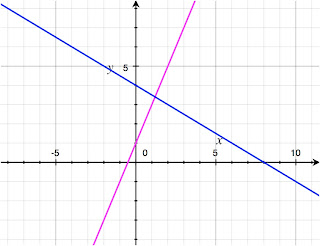# What is Perpendicularity?

"Perpendicular" is the term used in mathematics to describe two lines that intersect at right angles.  I recently introduced this concept in a separate post about the definition of perpendicular lines, but I thought it might be interesting to go into a little bit more detail about this rudimentary and familiar math concept.  For students who are just learning about graphing lines for the first time, this is undoubtedly sufficient, but for those more familiar with the concept, this post might provide additional insight.

I opened above by stating that we are talking about two intersecting lines that form a 90 degree angle with each other, though this definition can and should be expanded.   Though technically correct, there is more to the concept of "perpendicularity," as it's called when talking about this subject.  More correctly, this term applies to not only lines (and line segments), but planes (surfaces, not airplanes!) as well.  At first this may sound advanced, but if you think about it, it is completely obvious. If you stack two books together in a perpendicular arrangement, you essentially are viewing the intersection of two planes.  Lines are drawn on paper, but planes are like the three-dimensional versions that have depth., and you can readily find examples in real life of things that are at 90 degrees to each other.

That leads me to a second point about perpendicular lines and planes.  I have said that they intersect at 90 degrees, which is true again, but more accurately and mathematically you can say that the lines form two "congruent adjacent angles" (Wikipedia link).  This means that if you look at the perpendicular intersection in a T-shape, you see two angles next to each other (adjacent) that are the same (congruent).  And by the rules of geometry, since the angles along a straight line must add up to 180 degrees, this obviously means that the intersection must be composed of 90 degree angles.  Furthermore, if the lines extend through each other, the rules of geometry state that all angles around a point must sum up to 360 degrees, so again we have 90 degrees for each - four right angles.Perpendicular linesPerpendicular planes

Here's some additional information that you might find interesting.  The term "perpendicular" itself can be an adjective describing the lines, as in "the perpendicular lines are written in red ink."   Alternatively, it can be the noun, as in "the perpendicular to the ground rises to the sky."   Sorry, those examples aren't very creative!  Also, another word for perpendicular is "orthogonal," which can be used the same way to describe right angle intersections.  "Orthogonality"comes from the Greek for "straight angle" and refers to lines and planes at ninety degree angles, much like "perpendicularity."

I know these math definitions probably aren't going to make solving your graphing problems any easier, but I thought that it was good information to know!  It's a very important but basic concept that is introduced very early in math education, so hopefully I have explained it simply enough even for beginners to grasp.  Please click the +1 button below to share my post, and you can even tweet about my site if you like it!